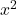## The length of a rectangle is 6 meters more than its width. The area of the rectangle is 114 square meters. Which of the following quadratic

Question

The length of a rectangle is 6 meters more than its width. The area of the rectangle is 114 square meters. Which of the following quadratic equations represents the area of the rectangle? Suppose x is the width of the rectangle. x 2-6x – 114 = 0​

A) x^2-6x-114=0

B) x^2-6x+114=0

C) x^2+6x+114=0

D) x^2+6x-114=0

in progress 0
2 months 2021-07-30T13:05:44+00:00 1 Answers 11 views 0

last one

Step-by-step explanation:

x = width

x+6 = length

Area = length times width

x(x + 6) =+ 6x+ 6x = 114   (subtract 114 from both sides)+ 6x -114 = 0